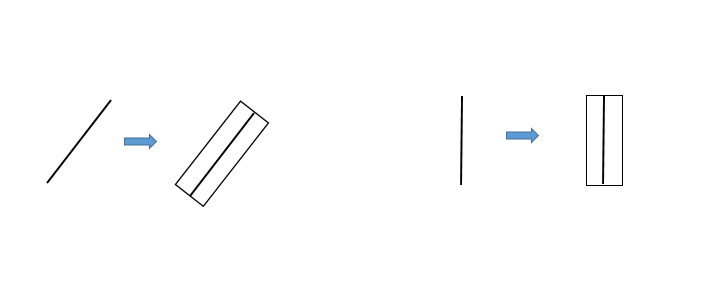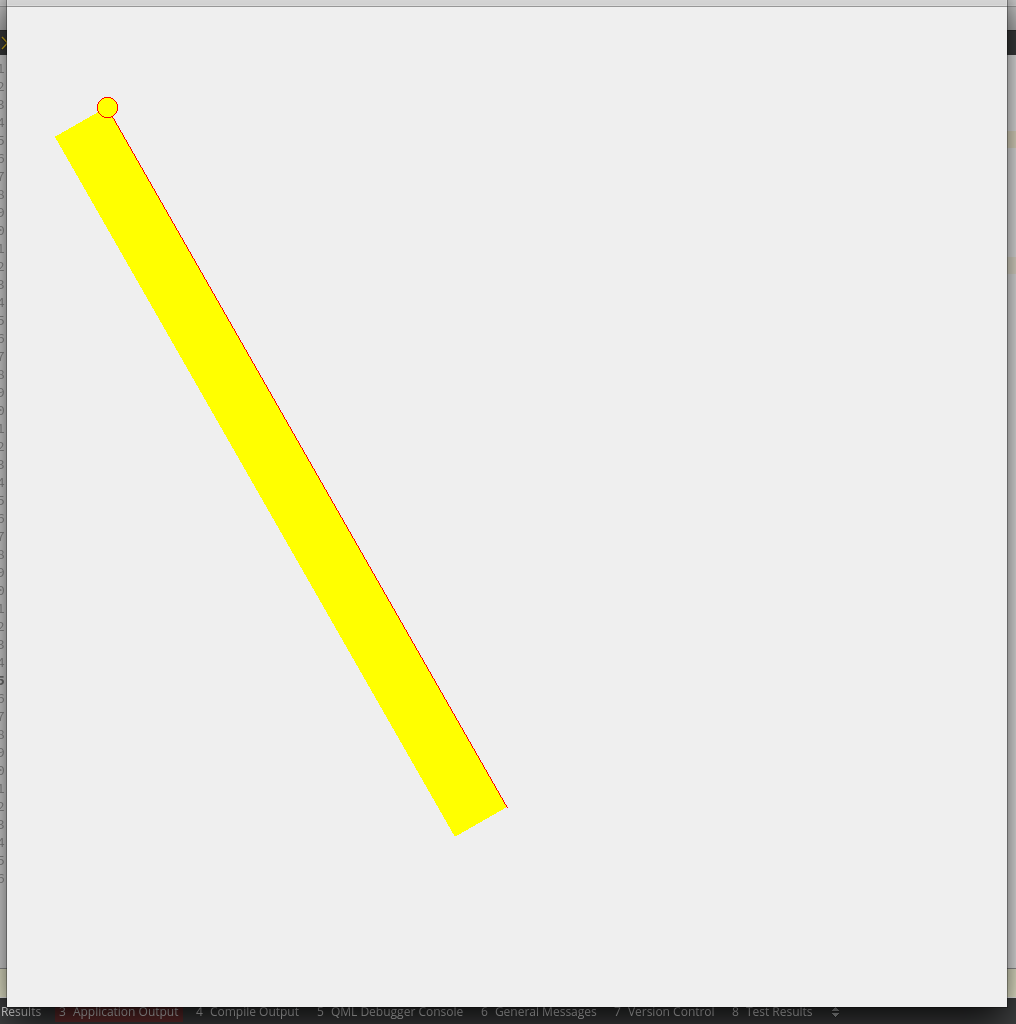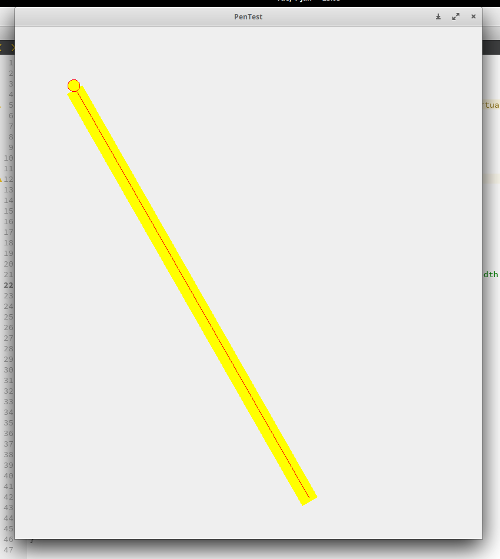# Draw a rectangle around a line

• I am trying to draw a "bounding box" around a line (basically I want a rectangle around a line so that the line cuts the rectangle in half). The line can have any angle and my rectangle always needs to be around it. Kind of like this picHere is my attempt in code:

``````#include <QApplication>
#include <QPainter>

class MyWidget : public QWidget{
public:
MyWidget(){

}

protected:
void paintEvent(QPaintEvent* event) override{
QPainter painter(this);
auto l1 = QPointF(100,100);
auto l2 = QPointF(500, 800);

auto w = 30; // rectangle width
QLineF line = QLineF(l1, l2);

QRectF rect = QRectF(0, 0, line.length(), w); // the rectangle will have the same width as the line's length
painter.translate(line.p1()); // set the first point of the line as the origin
painter.rotate(-line.angle()); // rotate the rectangle

rect.setWidth(line.length());
rect.setHeight(w);

painter.setPen(Qt::NoPen);
painter.setBrush(Qt::yellow);
painter.drawRect(rect);

painter.resetTransform();
painter.setPen(Qt::red);
painter.drawLine(line); // draw the line
painter.drawEllipse(l1, 10,10); // draw the origin
}
};

int main(int argc, char *argv[])
{
QApplication app(argc, argv);
MyWidget widget;
widget.resize(QSize(1000,1000));
widget.show();
return app.exec();
}

``````

The result is the followingAs you can see the line is not in the middle of the rectangle but on its top edge, how can I solve this?

• QLineF line = QLineF(l1, l2);

You need to offset the origin of your rect:

``````...
QLineF line = QLineF(l1, l2);
QPointF prect = line.p1();
prect.setX(prect.x()+w/2); // or set Y, not sure which
...
painter.translate(prect); // before drawing rect, keep line translate the same
``````

• QPointF prect = line.p1();
prect.setX(prect.x()+w/2); // or set Y, not sure which

I tried this (setting y and x), with setting x I get something close to what I need, but the line is not quite in the middle (but more 1/4 from the top), but with setting y the rectangle doesn't even contain the line.

• What happens if you do rotation first and then translate?

• @fcarney with rotation first and then translate is even worse, the rectangle gets moved perpendicularly to the line in the bottom left direction.

EDIT: there was a mistake in my code where I set the width of the rectangle to `w*2` . I corrected that. Now with

``````        QLineF line = QLineF(l1, l2);
QPointF prect = line.p1();
painter.translate(prect);
``````

I get something close to what I want (but not quite):